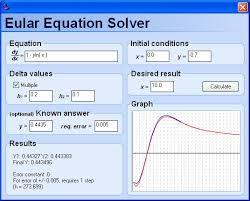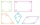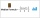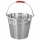# Simple equation

Solve the following simple equation:

2. (4x + 3) = 2-5. (1-x)We will be pleased if You send us any improvements to this math problem. Thank you!Tips to related online calculators
Do you have a linear equation or system of equations and looking for its solution? Or do you have a quadratic equation?

## Related math problems and questions:

• Equation 25Solve following simple equation: 3/4(x+5)=1/2(x+9)
• EquationSolve equation and check the result: 1.4x - 3/2 + x - 9,8 = x + 0,4/3 - 7 + 1,6/6
• Equation 29Solve next equation: 2 ( 2x + 3 ) = 8 ( 1 - x) -5 ( x -2 )3 3/4 + 2 3/5 + 5 1/2 Show your solution.
• Fraction of a NumberIf 1/2 of 1/3 of 1/4 of 1/5 of a number is 2.5. what is the number?
• Three digits number 2Find the number of all three-digit positive integers that can be put together from digits 1,2,3,4 and which are subject to the same time has the following conditions: on one positions is one of the numbers 1,3,4, on the place of hundreds 4 or 2.
• Simple equation 324 = n • 27, solve for n
• Find unknown 2Find unknown denominator: 2/3 -5/? = 1/4
• Fraction + eqSolve following simple equation with fractions: -5/6(8+5b) = 75 + 5/3b
• The perimeter 2The perimeter of the quadrilateral a = 1m b = 14/5m c = 2 3/10m d = 1 4/5m?
• Median or middleThe number of hours of television watched per day by a sample of 28 people is given below: 4, 1, 5, 5, 2, 5, 4, 4, 2, 3, 6, 8, 3, 5, 2, 0, 3, 5, 9, 4, 5, 2, 1, 3, 4, 7, 2, 9 What is the median value?
• Simple equation 5Solve equation with fractions: X × 3/8 = 1/2
• Find xSolve: if 2(x-1)=14, then x= (solve an equation with one unknown)
• Reciprocal equation 3Solve reciprocal equation: 1/2 + 2/3=1/x
• Gallons and quarts5 gallons 2 quarts − 1 gallon 3 quarts = (Hint: 4 quarts equals 1 gallon. )
• Eq1Solve equation: 4(a-3)=3(2a-5)
• LCD 2The least common denominator of 2/5, 1/2, and 3/4